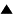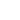# The Socio-technological Singularity

Although acceleration and complexity have made most concrete developments impossible to predict, large scale, statistical factors, such as wealth, life expectancy, intelligence, productivity, speed of information transmission and speed of information processing, increase in a surprisingly regular way. This makes it possible to extrapolate their development into the future. Most of these growth processes are exponential, characterized by a constant doubling period, or a constant increase in percentage per year. This means that the underlying growth mechanism is stable, producing a fixed number of new items for a given number of existing ones.

However, some processes grow even more quickly. For example, population growth in percentage per year is much larger now than it was a century ago. This is because medical progress has augmented the gap between the percentage of births and the percentage of deaths per year. If the growth of the world population over the past millenia is plotted out, the pattern appears to be hyperbolic rather than exponential. Hyperbolic growth is characterized by the fact that the inverse of the increasing variable (e.g. 1 divided by the total population) evolves according to a straight line that slopes downward. When the line reaches zero, this means that the variable (world population in this case) would become equal to 1 divided by zero, which means infinity. This is essentially different from an exponential growth process, which can never reach infinity in a finite time.Fig. 1: a hyperbolic growth process, where the growing number becomes infinite in the singular point, while its inverse (1 divided by the number) becomes zero.

If the line describing the inverse of world population until recently is extended into the future, we see that it reaches zero around the year 2035. Of course, such an extrapolation is not realistic. It is clear that world population can never become infinite. In fact, population growth is slowing down at this moment. However, that slowdown itself is a revolutionary event, which breaks a trend that has persisted over all of human history.

In mathematics, the point where the value of an otherwise finite and continuous function becomes infinite is called a "singularity". Since traditional mathematical operations, like differentiation, integration and extrapolation, are based on continuity, they cannot be applied to this singular point. If you ask a computer to calculate the value of one divided by zero it will respond with an error message. A singularity can be defined as the point where mathematical modelling breaks down. The inside of a "black hole" is an example of a singularity in the geometry of space. No scientific theory can say anything about what happens beyond its boundary. We can only postulate that different laws will apply inside, but these laws remain forever out of sight. Similarly, the Big Bang, the beginning of the universe, is a singularity in time, and no amount of extrapolation can describe what happened before this singular event.

The mathematician and science fiction writerVernor Vinge hasproposed that technological innovation is racing towards a singularity. Scientific discovery appears as an exponentially growing process with a doubling period of about 15 years. However, the doubling period is diminishing because of increasingly efficient communication and processing of the newly derived knowledge. The rate of growth is itself growing. This makes the process super-exponential, and possibly hyperbolic. Vinge would argue that at some point in the near future the doubling period would reach zero, which means that an infinite amount of knowledge would be generated in a finite time. At that point, every extrapolation that we could make based on our present understanding would become meaningless. The world will have entered a new stage, where wholly different rules apply. Whatever remains of humanity as we know it will have changed beyond recognition.

Some data supporting this conjecture can be found in a chart provided by Peter Peeters, in which the time elapsed between a dozen fundamental discoveries and their practical application is plotted against the year in which the invention was applied. The graph shows a downward sloping line, which reaches zero in the year 2000. These are just a few data points, and the implication that inventions will be applied virtually instantaneously after 2000 does not yet mean that scientific progress will be infinite. Vinge himself would situate the date of the singularity between 2010 and 2040. His reasoning is based on the accelerating growth of computer-aided intelligence. Rather than considering the IQ of an isolated individual, he would look at the team formed by a person and computer. According to Vinge, a PhD armed with an advanced workstation should already be able to solve all IQ tests ever devised. Since computing power undergoes a rapid exponential growth, we will soon reach the stage where the team (or perhaps even the computer on its own) would reach superhuman intelligence. Vinge defines this as the ability to create even greater intelligence than oneself. That is the point at which our understanding, which is based on the experience of our own intelligence, must break down.

A related reasoning was proposed by Jacques Vallée. Extrapolating from the phenomenal growth of computer networks and their power to transmit information, he noted that at some point all existing information would become available instantaneously everywhere. This is the "information singularity".

These models should not be taken too literally. They are metaphors, proposed to stimulate reflection. It does not make much sense to debate whether "the Singularity", if such a thing exists, will take place in 2029 or in 2035. Depending on the variable you consider most important, and the range over which you collect data points, you may find one date in which infinity is reached, or another, or none at all. Even if nothing as radical as a "New Age" could be predicted, the zero point method is certainly useful to attract the attention to possible crisis points, where the dynamics of change itself are altered. Peeters has used this method with some success to "predict" historical crises, such as the First World War and the 1930 and 1974 economic recessions. (The Second World War, strangely enough, did not seem to fit the model...).

The point to remember, however, is that abstract, non-material variables, such as intelligence, information, or innovation, aren't subjected to the same "limits to growth" which characterize the exhaustion of finite resources. Such variables could conceivably reach values which for all practical purposes may be called "infinite". Several parallel trends show a hyperbolic type of acceleration which seems to reach its asymptote (the point of infinite speed) somewhere in the first half of the 21st century. This does not mean that actual infinity will be reached, only that a fundamental transition is likely to take place. This will start a wholly new mode of development, governed by laws which we cannot as yet guess.

References:

 HomeMetasystem Transition TheoryThe Future of Humanity Up Prev.Next Down